D10, D100,D1000 Dice

 D10D10 (0-9) 0=10 (1-10) D10 (00-90)00=100 D10 (000-9000) 000=1000 D10 (0000-90000) 0000=10000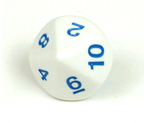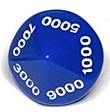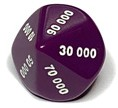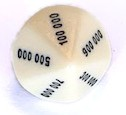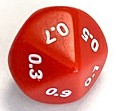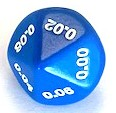How to do D100

 2xD10 (0-9)in different colorsone color for the Tenand the other for the unit Ex. 0 for Ten and 5 for unit = 52 for Ten and 7 for unit = 27 00 = 100 1xD10 (0-9) and 1xD10 (00-90)Ex.10 and 5 = 1590 and 5 = 9500 and 0 = 100 1Xd100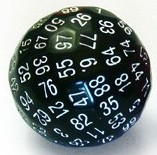How to do D1000

 3xD10 (0-9)in different colors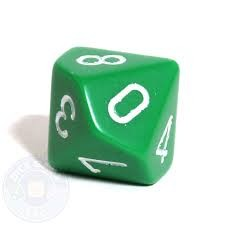one for the hundred other color for the Tenand the other for the unit Ex. 0 and 4 and 8 = 48 1 and 5 and 9 = 1599 and 9 and 9 = 999000 = 1000 2xD10 (0-9)in different colors and 1xD10 (00-90)Ex. 5 and 10 and 5 = 5150 and 90 and 5 = 950 and 00 and 0 = 1000 1xD10 (0-9) and 1xD10 (00-90)and 1xD10 (000-900)Ex. 000 and 20 and 0 = 20100 and 50 and 5 = 155900 and 90 and 9 = 999000 and 00 and 0 = 1000 1xD10 (0-9) and 1Xd100Ex.0 and 99 = 995 and 25 = 5259 and 99 = 9990 and 100 = 10001 and 100 = 1002 and 100 = 2003 and 100 = 300 Etc.

10 millions Dice
D10000000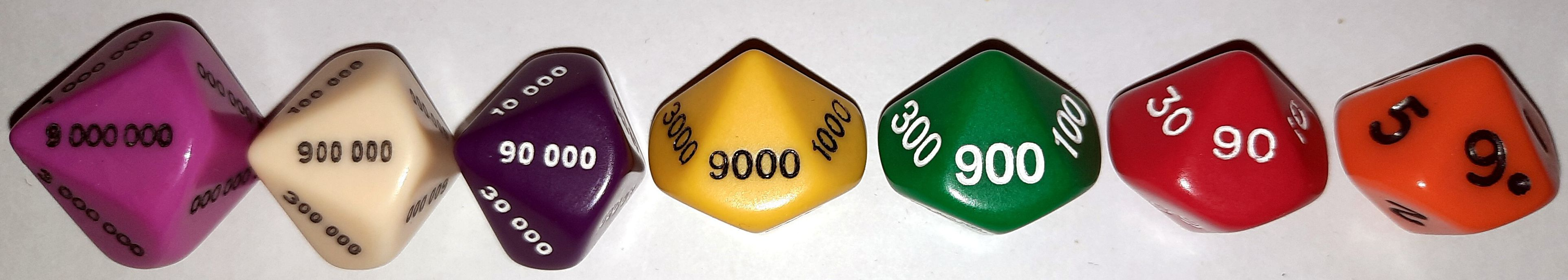D256 Hexadecimal die, 00 to FF , FF = 255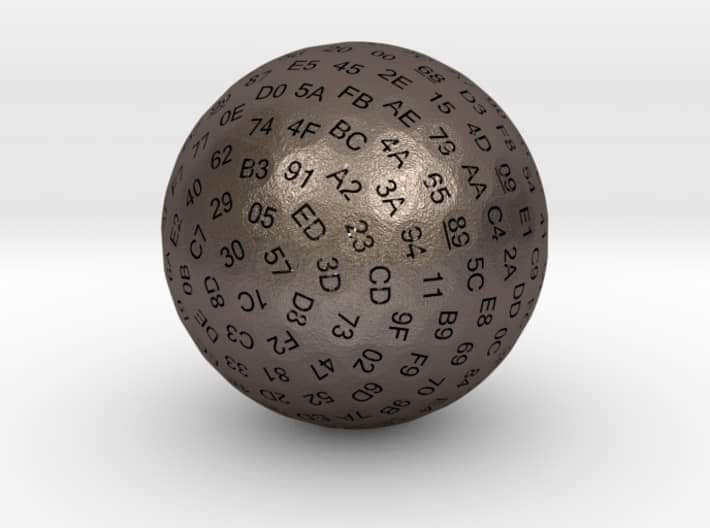Recherche personnalisée